# Domain‑Specific Languages: Part 2

Episode #27 • Aug 27, 2018 • Subscriber-Only

We finish our introduction to DSLs by adding two new features to our toy example: support for multiple variables and support for let-bindings so that we can share subexpressions within a larger expression. With these fundamentals out of the way, we will be ready to tackle a real-world DSL soon!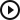Domain‑Specific Languages: Part 2
Introduction
00:05
Recap
01:51
Adding multiple variables to our DSL
04:23
Adding let bindings to our DSL
10:20
What’s the point?
18:03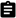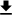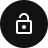### Introduction

In the last episode we gave a defined “domain specific languages”, also known as DSLs, as any language that is highly tuned to a specific task. Some popular examples are SQL, HTML, and even Cocoapods and Carthage. We also defined an “embedded domain specific language”, also known as an EDSL, as a DSL that is embedded in an existing language. The `Podfile` of Cocoapods is an example of this, because the `Podfile` is technically written in standard Ruby code.

We then began constructing a toy EDSL example in Swift from first principles. It modeled an arithmetic expression where only integers, addition, multiplication, and variables were allowed. We defined two interpretations of this DSL: one for evaluating the expression to get an integer from it once you do all the arithmetic, and also a printer so that we could represent the expression as a string. We also experimented a bit with transforming the DSL abstractly, such as performing some basic simplification rules on an expression, such as factoring out common values.

This time we are going to add two very advanced features to our DSL: the ability to support multiple variables, and the ability to introduce let-bindings, which allows us to share expressions within our DSL. It’s kinda strange and cool.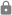This episode is for subscribers only.

### Subscribe to Point-Free

Access this episode, plus all past and future episodes when you become a subscriber.

See plans and pricing

### Exercises

1. Implement an inliner function `inline: (Expr) -> Expr` that removes all `let`-bindings and inlines the body of the binding directly into the subexpression.

2. Implement a function `freeVars: (Expr) -> String` that collects all of the variables used in an expression.

3. Define an infix operator `.=` to mimic `let`-bindings. At the call site its usage might look something like: `("x" .= 3)("x" * 2 + 3)`, where we are using the infix operators `*` and `+` defined in the exercises of the last episode.

4. Update `bind` to take a dictionary of bindings rather than a single binding.

5. In this exercise we are going to implement a function `D: (String) -> (Expr) -> Expr` that computes the derivative of any expression you give it. This may sound scary, but we’ll take it one step at a time!

• Let’s start simple. The signature `D: (String) -> (Expr) -> Expr` represents the concept of taking the derivative of an expression with respect to some variable, specified by the `String` argument. Write down the signature of this function, and call the string argument `variable`. This string is the variable with respect to which you will be differentiating. Also implement the body as a closure that takes a single argument (the expression), and inside the closure implement the `switch` over that argument while leaving all the cases unimplemented.

• Derivatives have the simple property that they annihilate constants: `D(1) = 0`, `D(-1) = 0`, `D(2) = 0`, i.e. the derivate of any constant is zero. Use this fact to implement the `.lit` case in the `switch` you defined above.

• Derivatives also have a simple property for variables. The derivative of a variable with respect to that variable is simply `1`, and the derivative of any variable with respect to any other variable is `0`. Use this fact to implement the `.var` case in the `switch` you defined above.

• Derivatives have the wonderful property that they distribute over addition: `D(f + g) = D(f) + D(g)`, i.e. the derivative of a sum is the sum of the derivatives. Use this fact to implement the `.add` case in the `switch` you defined above.

• Derivatives have a slightly more complicated relationship with multplication. It is not true that derivatives distribute over multiplication, but they do something close: `D(f * g) = D(f) * g + f * D(g)`. Use this fact to implement the `.mul` case in the `switch` you defined above.

• Finally, derivatives have an even more complicated relationship with let-bindings: `D(f >>> g) = D(f) * (f >>> D(g))`, where here we are using `>>>` as a shorthand to represent the idea that let-bindings are essentially function composition. It’s a lot to take in, but what this is saying is that you take the derivative of the expression you are binding `D(f)`, multiply that with the derivative of the subexpression that uses the binding `D(g)` pre-composed with the binding `f >>> D(g)`. Use this fact to implement the `.bind` case in the `switch` you defined above.

If you can solve these exercises, you’ve essentially done a semester’s worth of calculus!

6. Use the `D` function defined above to differentiate some expressions.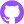0027-edsls-pt2